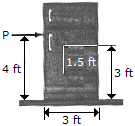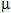# Engineering Mechanics - Friction - Discussion

### Discussion :: Friction - General Questions (Q.No.4)

4.The refrigerator has a weight of 200 lb and a center of gravity at G. Determine the force P required to move it. Will the refrigerator tip or slip? Take= 0.4.

 [A]. P = 75 lb Slips [B]. P = 80 lb Tips [C]. P = 80 lb Slips [D]. P = 75 lb Tips

Explanation:

No answer description available for this question.

 Shiva said: (Nov 21, 2011) How could it be? It is 80lb Tips

 Svenu said: (Sep 7, 2012) It could 80 lb why 75 can any one tell me

 Naveen Goud said: (Mar 13, 2013) Take the moments of p&weight about other end of bottom point then p*4+(200*1.5)=0 p=75 &tips about that point.

 Adla said: (Jul 18, 2014) Can anyone give me the working out for this question?

 Qahtan said: (Jul 1, 2015) tan (theta) = (meu_k) = 1.5/h; (meu_k) = 0.4. 0.4 = 1.5/h ; h = 3.75. Where h = 4 (meu_k) = 1.5/4 = 0.375. Then at (meu_k) = 0.4 the refrigeration is tips. And at (meu_k) = 0.375 the refrigeration is slips. Sum moments about other end equal to zero. P*4-(200*1.5) = 0; p = 200*1.5/4; p = 75 lb tips.

 Shashi Kumar said: (Nov 3, 2016) Please, tell me the right answer with proper explanation.

 Arun Kumar said: (Jan 4, 2017) Answer C is also possible when we take equilibrium condition. As we considering friction at the base & normal reaction. Its also correct.

 Prahlad said: (Oct 2, 2017) It means p is the value in which body are statics or move then the answer is D.

 Prahlad Bhardwaj said: (Oct 2, 2017) P =200lb*1.5/4 =75ans.